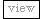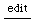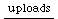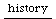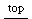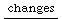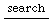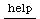## put not understood

```//how to create this error:

a = Pbind(\amp, 0.0).asStream;
a.next.postln;

//error:

ERROR:
Message 'put' not understood.

RECEIVER:
nil
ARGS:
Instance of Array {    (08CE7910, gc=8C, fmt=01, flg=00, set=01)
indexed slots 
0 : Symbol 'amp'
1 : Float 0
}
CALL STACK:
Object::doesNotUnderstand
arg this = nil
arg selector = 'put'
arg args = 
< FunctionDef in Method Pbind::asStream >
arg i = 0
var name = 'amp'
var stream = 0.000000
var streamout = 0.000000
Integer::forBy
arg this = 0
arg endval = 1
arg stepval = 2
arg function =
var i = 0
var j = 0
< FunctionDef in Method Pbind::asStream >
arg inval = nil
var sawNil = false
< closed FunctionDef >  (no arguments or variables)
Interpreter::interpretCmdLine
arg this =
var function =
Process::interpretCmdLine
arg this =

//solution:

an eventstream always puts the values from each stream
of the Pbind into the protoevent, which is nil here.

a = Pbind(\amp, 0.0).asStream;
a.next(Event.new).postln;
or:

a = Pbind(\amp, 0.0).asEventStream(Event.new);
a.next.postln;

```

### Links to this Page

• enter. last edited on 29 January 2003 at 10:46 pm by 203.14.169.19
• Error Messages last edited on 16 November 2003 at 6:30 pm by max2-247.dialin.uni-hamburg.de